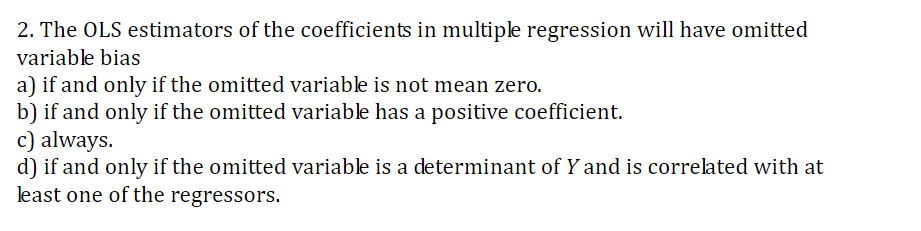# 2. The OLS estimators of the coefficients in multiple regression will have omittedvariable biasa) if and only if the omitted variable is not mean zero.b) if and only if the omitted variable has a positive coefficient.c) alwaysd) if and only if the omitted variable is a determinant of Y and is correlated with atleast one of the regressors.

Question
1 viewshelp_outlineImage Transcriptionclose2. The OLS estimators of the coefficients in multiple regression will have omitted variable bias a) if and only if the omitted variable is not mean zero. b) if and only if the omitted variable has a positive coefficient. c) always d) if and only if the omitted variable is a determinant of Y and is correlated with at least one of the regressors. fullscreen
check_circle

Step 1

Omitted variable bias:

The bias in the ordinary least square estimator that occurs as a resul...

### Want to see the full answer?

See Solution

#### Want to see this answer and more?

Solutions are written by subject experts who are available 24/7. Questions are typically answered within 1 hour.*

See Solution
*Response times may vary by subject and question.
Tagged in

### Other